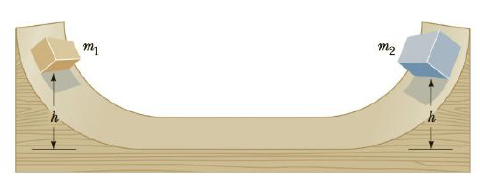Chapter 6, Problem 70AP

Chapter
Section
Textbook Problem

Two blocks of masses m1 = 2.00 kg and m2 = 4.00 kg are each released from rest at a height of h = 5.00 m on a frictionless track, as shown in Figure P6.70, and undergo an elastic head-on collision, (a) Determine the velocity of each block just before the collision, (b) Determine the velocity of each block immediately after the collision, (c) Determine the maximum heights to which m1 and m2 rise after the collision.Figure P6.70

(a)

To determine
The velocity of each block just before the collision.

Explanation

Given info:

The acceleration due to gravity is 9.80m/s2 .

The height of the blocks is 5.00m

Explanation:

The Formula to calculate the conservation of mechanical energy,

(K.E+P.Eg)i=(K.E+P.Eg)012mvi2+0=0+mgh012mvi2=mgh0

• (K.E+P.Eg)0 is the total energy of the block before collision
• (K.E+P.Eg)i is the total energy of the actor initially
• h0 is the height
• m is the mass
• g is the acceleration due to gravity
• vi is the speed

Rearranging the formula gives the speed as,

vi=2gh0 (I)

The speeds of the blocks are similar in magnitude and opposite to each other

(b)

To determine
The velocity of each block immediately after the collision.

(c)

To determine
The height at which each block reaches after the collision.

Answer: The height at which first block reaches after collision is  13.9m and the height at which second block reaches after collision is 0.556m .

Still sussing out bartleby?

Check out a sample textbook solution.

See a sample solution

The Solution to Your Study Problems

Bartleby provides explanations to thousands of textbook problems written by our experts, many with advanced degrees!

Get Started